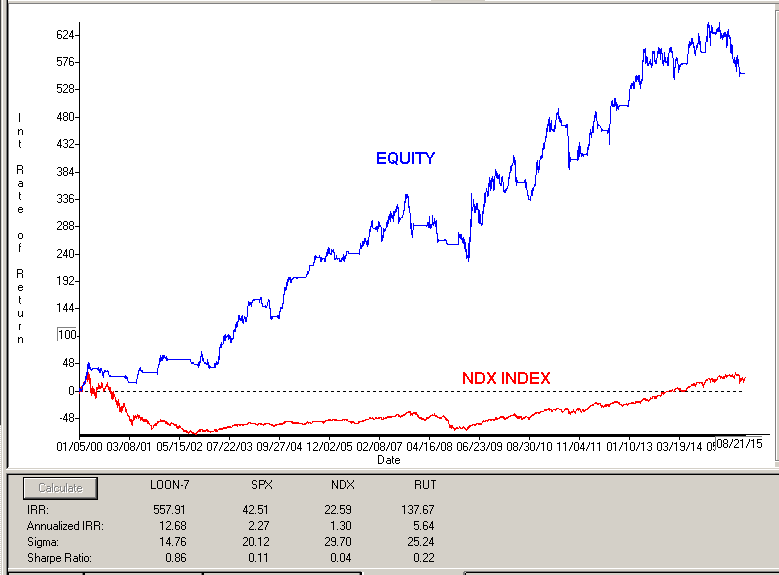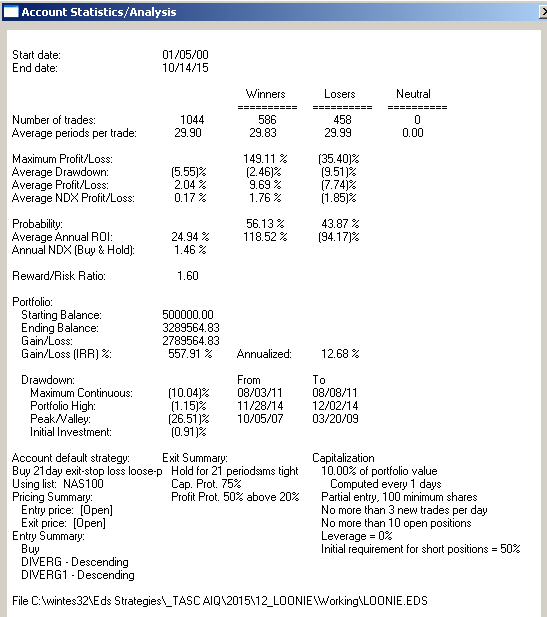# Markos Katsanos’s “Trading The Loonie”

Here is some code for use in AIQ based on Markos Katsanos’s article in Stocks and Commodities, “Trading The Loonie.”
The code I am providing contains both the divergence indicator and a long-only trading system for the NASDAQ 100 list of stocks. Rather than trading forex, I wanted to try the divergence idea and the author’s entry rules on the NASDAQ 100 stocks.
The stocks are traded long using the author’s entry rules with two of the parameters adjusted as shown at the top of the code file. The exit has been changed completely to use a profit protect (protect 50% of profits once a 20% profit is reached), a stop-loss (protect 75% of capital), and a time-stop exit (exit after 21 days).
I used the NASDAQ 100 index (NDX) in place of the crude oil futures. The assumption is that since the stocks on the list are all in the NDX, they would generally be correlated to the index. The author’s entry rule filters out those with a negative correlation to the index. Note that I changed the minimum correlation from a -0.4 to 0.0. In addition, I found that increasing the minimum divergence from 20 to 2,000 increased the Sharpe ratio and decreased the maximum drawdown without affecting the annualized return.
Figure 6 shows the equity curve versus the NASDAQ 100 index for the period 1/5/2000 to 10/14/2015. Figure 7 shows the metrics for this same test period. The system clearly outperformed the index.FIGURE 6: AIQ. Here is a sample equity curve for the modified divergence system versus the NASDAQ 100 index for the period 1/5/2000 to 10/14/2015.FIGURE 7: AIQ. Here are the metrics for the modified system and the test settings.
```!TRADING THE LOONIE
!Author: Markos Katsanos, TASC December 2015
!coded by: Richard Denning 10/17/15

!Set parameters:
Define Len        20. !Default is 20
Define F1          2. !Default is 2
Define F2          4. !Default is 4
IDX is         "NDX". !NASDAQ 100 index
IDXsLen is        40. !Default is 40
minDIVERG is    2000. !Default is 20
minROC is          0. !Default is 0
minCorrel is     0.0. !Default is -0.4

!Close percent relative to BB band width for stock:
Variance is Variance([close],Len).
StdDev is Sqrt(Variance).
SMA is simpleavg([close],Len).
stkBB is 1+([close]-SMA+F1*StdDev)/(F2*StdDev).

!Close percent relative to BB band width for index:
IDXc is tickerUDF(IDX,[close]).
VarianceIdx is Variance(IDXc,Len).
StdDevIDX is Sqrt(Variance).
SMAidx is simpleavg(IDXc,Len).
idxBB is 1+(IDXc-SMAidx+F1*StdDevIDX)/(F2*StdDevIDX).

DIVERG is (idxBB-stkBB)/stkBB*100.     !PLOT AS CUSTOM INDICATOR
DIVERG1 is valresult(DIVERG,1).
ROC2 is ([close]/val([close],2)-1)*100.
ROC3 is ([close]/val([close],3)-1)*100.
ROC3idx is tickerUDF(IDX,ROC3).
IDXsma is simpleavg(IDXc,IDXsLen).
IDXsma2 is valresult(IDXsma,2).
HHVdiverg is highresult(DIVERG,3).

Setup1 if highresult(DIVERG,3) > minDIVERG.
Setup2 if DIVERG < valresult(DIVERG,1).
Setup3 if ([close]/val([close],2)-1)*100 > minROC.
Setup4 if IDXsma > valresult(IDXsma,2).
Setup5 if pCorrel > minCorrel.

Setup2 and
Setup3 and
Setup4 and
Setup5.

LongExit1 if MACDsigMACD,1) and
Stoch > 85.
LongExit2 if lowresult(DIVERG,3)=21 or [close] <= (1-0.25)*{position entry price}.

!Code to Calculate Pearson's R [for entry]:
! PeriodtoTest is the number of lookback days.
! IndexTkr is the Instrument that you which to compare your list to.
PeriodToTest is Len.
IndexTkr is IDX.
ChgTkr is ([open] / val([open],PeriodToTest)-1)*100.
ChgIdx is TickerUDF(IndexTkr,ChgTkr).
Alpha is ChgTkr - ChgIdx.

ValUDF is (([close]-[open])/[open]) * 100.
ValIndex is TickerUDF(IndexTkr, ValUDF).
ValTkr is ValUDF.
SumXSquared is Sum(Power(ValIndex,2), PeriodToTest).
SumX is Sum(ValIndex, PeriodToTest).
SumYSquared is Sum(Power(ValTkr,2), PeriodToTest).
SumY is Sum(ValTkr, PeriodToTest).
SumXY is Sum(ValTkr*ValIndex, PeriodToTest).
SP is SumXY - ( (SumX * SumY) / PeriodToTest ).
SSx is SumXSquared - ( (SumX * SumX) / PeriodToTest ).
SSy is SumYSquared - ( (SumY * SumY) / PeriodToTest ).

!Pearson's R and Pearson's Coefficient of Determination:
pCorrel is SP/SQRT(SSX*SSY).

!Code to Calculate Pearson's R [for exit]:
! PeriodtoTest is the number of lookback days.
! IndexTkr is the Instrument that you which to compare your list to.
PeriodToTestX is 3*Len.
IndexTkrX is IDX.
ChgTkrX is ([open] / val([open],PeriodToTestX)-1)*100.
ChgIdxX is TickerUDF(IndexTkrX,ChgTkrX).
AlphaX is ChgTkrX - ChgIdxX.

ValUDFX is (([close]-[open])/[open]) * 100.
ValIndexX is TickerUDF(IndexTkrX, ValUDFX).
ValTkrX is ValUDFX.
SumXSquaredX is Sum(Power(ValIndexX,2), PeriodToTestX).
SumXX is Sum(ValIndexX, PeriodToTestX).
SumYSquaredX is Sum(Power(ValTkrX,2), PeriodToTestX).
SumYX is Sum(ValTkrX, PeriodToTestX).
SumXYX is Sum(ValTkrX*ValIndexX, PeriodToTestX).
SPX is SumXYX - ( (SumXX * SumYX) / PeriodToTestX).
SSxX is SumXSquaredX - ( (SumXX * SumXX) / PeriodToTestX ).
SSyX is SumYSquaredX - ( (SumYX * SumYX) / PeriodToTestX ).

!Pearson's R and Pearson's Coefficient of Determination:
pCorrelX is SPX/SQRT(SSXX*SSYX).

!MACD code:
S is 12.
L is 25.
X is 9.

ShortMACDMA is expavg([Close],S).
LongMACDMA is expavg([Close],L).

MACD is ShortMACDMA-LongMACDMA.
SigMACD is expavg(MACD,X).

!Stochastic
StochLen is 30.
Stoch is 100 * (([Close]-LoVal([Low],StochLen)) /
(HiVal([High],StochLen) - LoVal([Low],StochLen))).

List if 1.

—Richard Denning